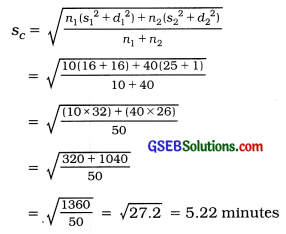# GSEB Solutions Class 11 Statistics Chapter 4 Measures of Dispersion Ex 4.6

Gujarat Board Statistics Class 11 GSEB Solutions Chapter 4 Measures of Dispersion Ex 4.6 Textbook Exercise Questions and Answers.

## Gujarat Board Textbook Solutions Class 11 Statistics Chapter 4 Measures of Dispersion Ex 4.6

Question 1.
The information regarding marks of the students of two classes of a school is given below. Find the combined standard deviation of the marks obtained by the students.

 Division A Division B No. of Students 50 60 Mean marks 60 48 Standard deviation 10 12

Class A:
n1 = No. of students = 50
1 = Average marks = 60
s1 = Standard deviation = 10

Class B:
n2 = No. of students = 60
2 = Average marks = 48
s2 = Standard deviation = 12

Combined mean of marks of students of Class A and Class B:
c = $$\frac{n_{1} \bar{x}_{1}+n_{2} \bar{x}_{2}}{n_{1}+n_{2}}$$
= $$\frac{(50 \times 60)+(60 \times 48)}{50+60}$$
= $$\frac{3000+2880}{110}=\frac{5880}{110}$$ = 53.45 marks

Combined standard deviation of marks of students of Class A and Class B:
d1 = x̄1 – x̄c = 60 – 53.45 = 6.55
∴ d12 = 42.9025

d2 = x̄2 – x̄c = 48 – 53.45 = 5.45
∴d21 = 29.7025
s1 = 10 ∴ s12 = 100: s2 = 12 ∴ s22 = 144Question 2.
The following information is available for two sections of a factory. Obtain the combined standard deviation of the production time.

 Section A Section B No. of workers 10 40 Mean production time per unit (minute) 25 20 Variance 16 25

Section A:
n1 = No. of workers =10
1 = Average production time per unit = 25
s11 = Variance =16

Section B :
n2 = No. of workers = 40
2 = Average production time per unit = 20
s22 = Variance = 25

Combine mean of production time per unit of Section A and Section B:
c = $$\frac{n_{1} \bar{x}_{1}+n_{2} \bar{x}_{2}}{n_{1}+n_{2}}$$
= $$\frac{(10 \times 25)+(40 \times 20)}{10+40}$$
= $$\frac{250+800}{50}=\frac{1050}{50}$$ = 21 minutes

Combined standard deviation of production time per unit of Section A and Section B :
d1 = x̄1 – x̄c = 25 – 21 = 4
∴ d12 = 16

d2 = x̄2 – x̄c = 20 – 21 = -1
∴d21 = 1

s1 = 4 ∴ s12 = 16: s2 = 5 ∴ s22 = 25